Home MATHEMATICS TOPIC 5: SEQUENCE AND SERIES ~ MATHEMATICS FORM 3

# TOPIC 5: SEQUENCE AND SERIES ~ MATHEMATICS FORM 3

1673
30
SHARESequences
The Concept of Sequence
Explain the concept of sequence
A Sequence is the arrangement of numbers or is a list of numbers following a clear pattern such that one number and the next are separated by comma (,).
Example: a1, a2, a3, a……………………..
NB: Each number found in a Series or Sequence is called a term.
Example 1
Find the next three terms in the following sequences.
1. 5, 8, 11, 14, 17,………………………………
2. 3, 7, 6, 10, 9, …………………………………
3. 1, 2, 4, 7, ………………………………………
4. 2, 9, 20, 35, …………………………………
Solution:
(a)You can see that each term is less to the next by 3.
So next three terms are (17+3),(17+3+3) and 17+3+3×3)
Which are 20, 23, and 26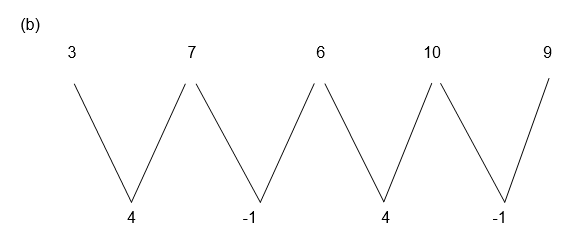Alternately add 4 and subs tract 1. The sequence then extends to 13, 12, 16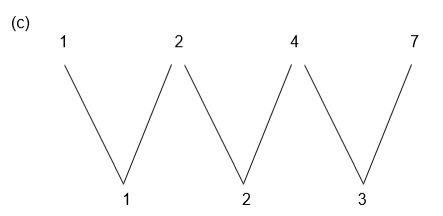We see that the difference is increasing by 1 each time. So the next three terms are 11, 16 and 22.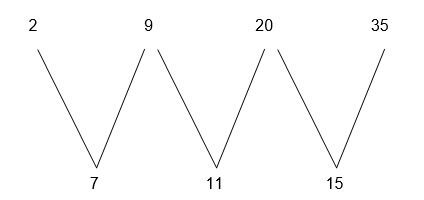The differences are increased by 4 each time, so the next three terms are 54, 77 and 104.
Example 2
Write down the first three terms in the sequences where the nth term is given by the formulae.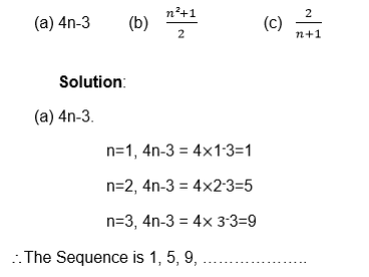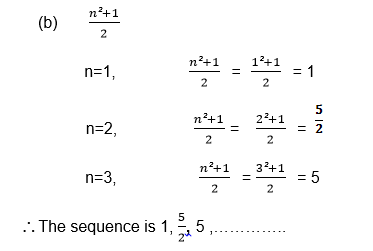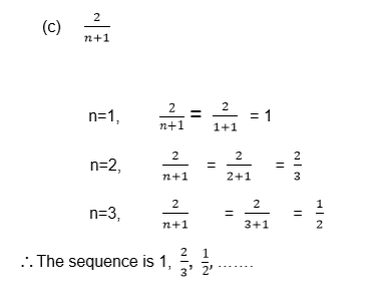Example 3
The kth term of a series is k+ 4
Find the sum of the first four terms in the series
Solution:
k=1, k2+4=12+4=5
k=2, k2+4=22+4=8
k=3, k2+4=32+4=13
k=4, k2+4=42+4=20
So the series is 5+8+13+20 and its sum is 46
Example 4
Find the nth term of the following sequences: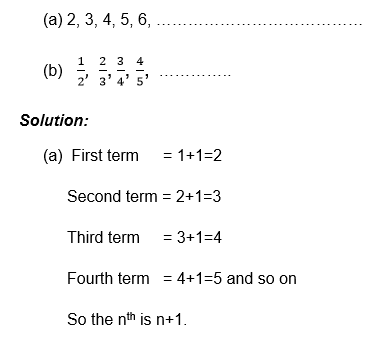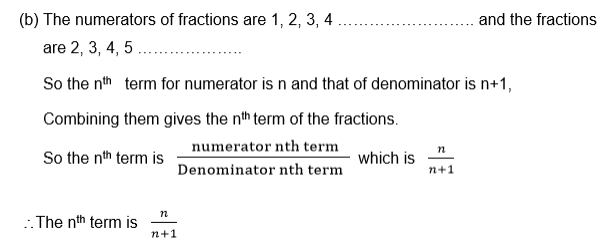Exercise 1
1. Write down the next three terms in the following sequences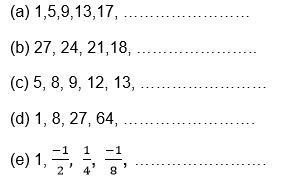2. Find the first three terms in the sequence:
1. 5n+2
2. 1-3k
3. n2+n+1
4. 2n
3. Find the sum of the first four terms of the series where the kth term is given by:
1. 5k+3
2. k3-1
3. 2k
4. Find the nth term of these sequences: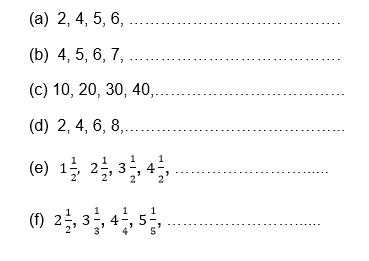An Arithmetic Progression (AP) and Geometric Progression (GP)
Identify an arithmetic progression (AP) and geometric progression (GP)
When
the series or sequence is such that between two consecutive terms there
is a difference which is fixed, then the series or sequence is called
an arithmetic progression (A.P)
The fixed difference (number) between two consecutive terms is called the common difference (d)
Example 5
In the sequence 4, 7, 19, 13, 16 there is a common difference which is
7-4=10-7=13-10=16-13=3.
So the common difference (d)=3.
Note that in arithmetic progression (A.P) the difference between two successive terms is always the same.
Sometimes
numbers may be decreasing instead of increasing, the arithmetic
sequence or series while terms decrease have a negative number as a
common difference.
Example 6
The common difference of the sequence 6, 4, 0, -2, …………………… is
4-6=2-4=0-2=-2-0=-2
So the common difference is –2.
In general if A1, A2, A3, A4, ……………………… An are the terms of the arithmetic sequence , then the common difference is ;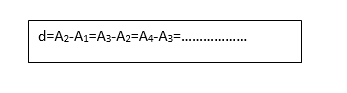Example 7
For each of the following sequences, find the common difference and write the next two terms.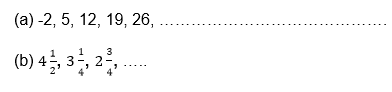Solution: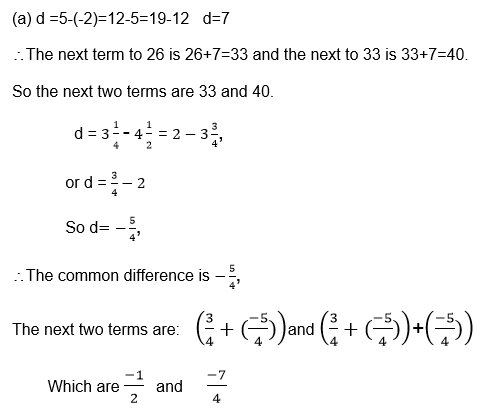Exercise 2
1. Find the common difference for each of the following sequence:
1. 11, 14, 17, 20, …………………………………
2. 2, 4, 6, 8, 10, ……………………………………
3. 0.1, 0.11, 0.111, 0.1111 , …… … … … … …
4. y, y+3, y+6, y+9, y+12, … …… … … … ……
2. State whetherthe following sequence are arithmetic or not:
1. 2, 5, 8, 11, 14, …………… ……………… ……
2. 1, 3, 4, 6, 7, 9, 10, ………………………………
3. y, y + x, y+2x, y+3x, … ………… ……
3. The temperatureat a mid day is 30c, and it falls by 20c each hour. Find the temperature at the end of the next four hours.
Geometric Progression (G.P).
When
the series or Sequence is such that between two consecutive terms there
is a ration which is fixed, then the series or sequence is called a
geometric progression (G.P)
The fixed ratio(number) between two successive terms is called the common ratio (r).
Example 8
In 2, 4, 8, 16, 32, … … …… … … …….
There is a common ration which is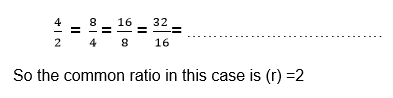Note that like in arithmetic progression (A.P), in geometric progression (G.P) the common ratio does not change.
Also
the terms may be decreasing instead of increasing, the geometric
sequence or series whose terms decrease have a positive common ratio
which is less than 1 for the progression with positive terms.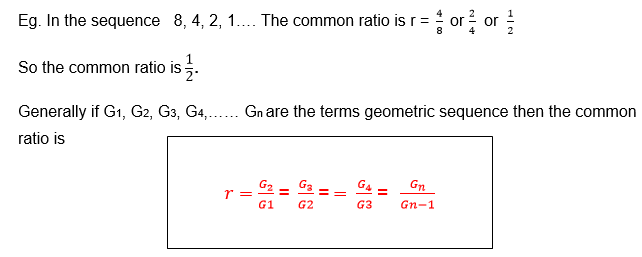Example 9
For each of the following sequence find the common ratio.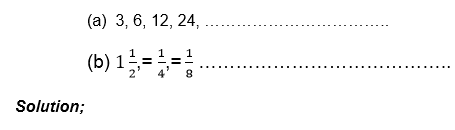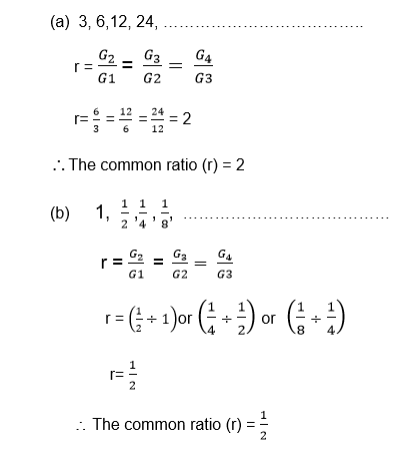Example 10
For the following geometric sequences, find the common ratio and write down the next two terms: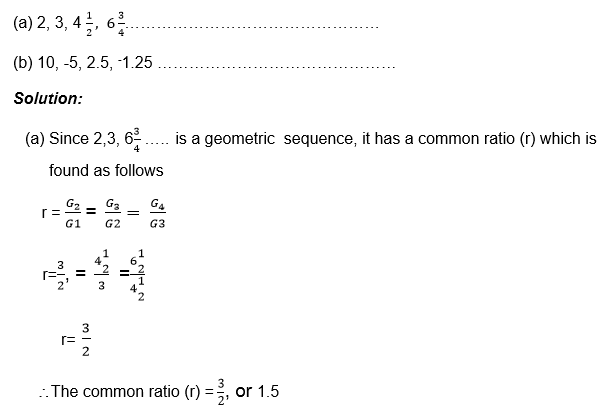The next term is found by multiplying the term considered to be the last term by the common ratio.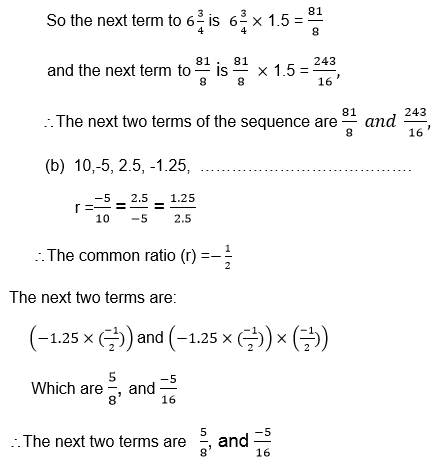Exercise 3
1. Which of the following sequences are geometric
1. 1, 2, 4, 8, 16, ……………………………………
2. 2, 6, 18, 54, 162, …………………………………
3. 1, -1,1,-1,1, ………………………………………
4. x2, 2x3, 4×4, 8x3…………………………………
5. 1, 2, 4, 7, 10, ………………………………………
6. 0.1, 0.2, 0.3, 0.4, 0.5, ……………………………
7. 3, 6, 9, 12,15, ……………………………………….
2. Find thecommon difference for each of the following geometric progressions (G.P)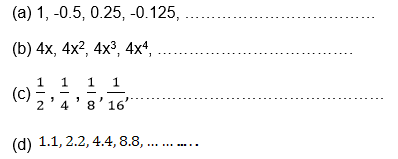3. Find thenext term of the sequence 2, 10, 50, 500,………………….
4.
The populationof a town is decreasing so that every year the population
declines by a quarter. If the population is originally 100,000. What
will it be after 5 years?
The General Term of an AP
Find the general term of an AP
If A1, A2, A3, …………………Aare the terms of an arithmetic sequence, then there is a common difference d which is given by
d = A2 – A1 = A3 – A2 = An – An – 1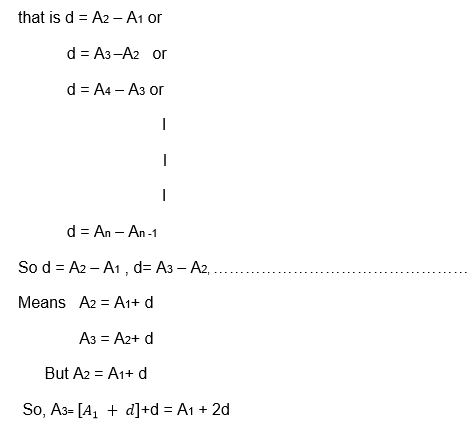But . A3 = A1 + 2d which means
A=[ A1+2d]+d
= A1 + 3d
Putting into consideration this pattern, it is true that
A= A1 + 4d
A= A1 + 5d
An = A1 + (n-1)d
Where An is the nth term
The nth term of the sequence with first term Aand common difference d is given by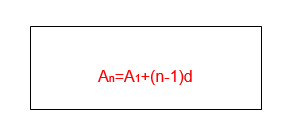Example 11
Find theformula for the nth term of the sequence 8 , 9.5, 11, 12.5, 14, 15.5,……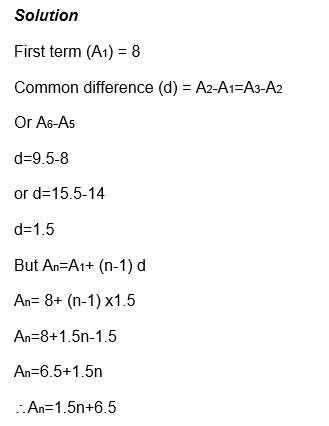Note that the nth term gives every term in the sequence,
For example when n=3, you have A3=1.5×3+6.5=11
So A3=11 where 11 is given in the sequence above having the third position.
Therefore An shows the position of the term in sequence and of A1+(n-1)d gives the value of the term for any positive integer.
Example 12
The 5th term of an arithmetic sequence is 11, and the 8th term is 26. Find the first five terms.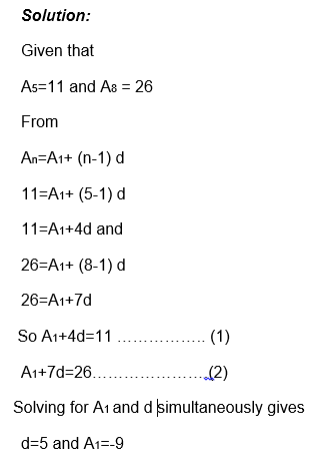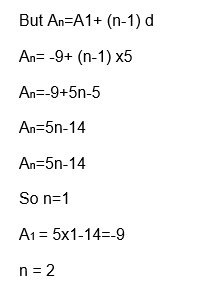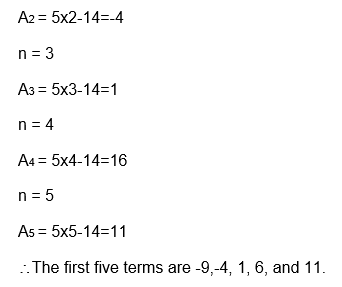Example 13
The 8th term of an arithmetic sequence is 9 greater than the 5th term, and the 10th term is 10 times the 2nd term. Find
1. The common difference (d)
2. 20th term.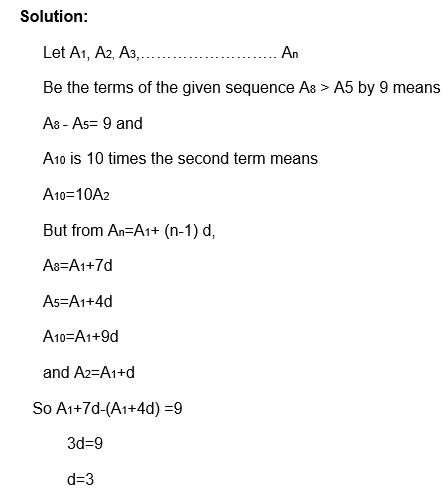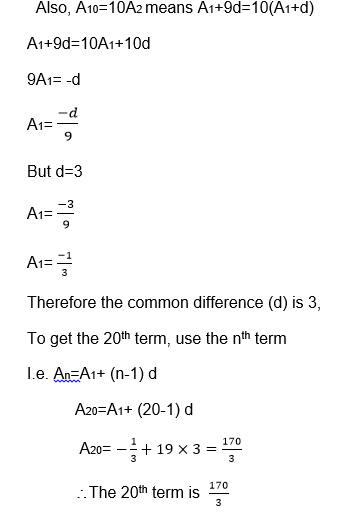The General Term of GP
Find the general term of GP
If G1, G2, G3,……………..Gn are the terms of a geometric sequence, then they have a common ratio (r) which is given byExample 14
Find the formula for the nth term of each of the following geometric sequence.
1. 2, 6, 18, 54 , ………………………………
2. 4,-2, 1, -0.5, 0.25 …………………………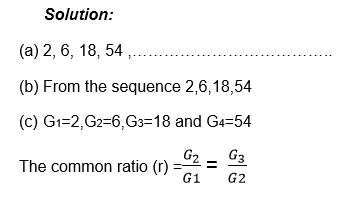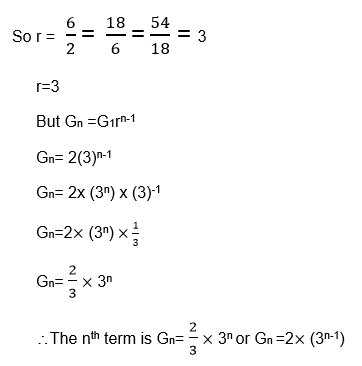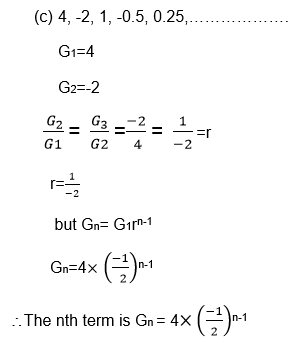Example 15
Considering that,Exercise 4
1. In the arithmetic sequence, the 17th term is 30 and 9th term is 42 find the first three terms.
2. In the Arithmeticsequence the third term 12 and the 9th term 24. Find the nth term of the sequence and use it to find the 15th term.
3. Find the15th term of the sequence 5, 10, 20, 40 ,…………………………
4.
A population isincreasing and every year it is multiplied by 1.03. If
it starts off at 10,000,000, what will it be after n years?
5. The first termof the geometric sequence is 7 and the common ratio is 4. What is the 9th term of this sequence?

1. Hi! This is kind of off subject but I need some assistance from an established blog. Is it difficult to establish your very own blog? I’m not extremely techincal however I can figure things out pretty quick. I’m considering establishing my very own but I’m. not exactly sure where to start. Do you have any points or pointers? Thanks.

2. This info is invaluable. When can I find out more? asmr 0mniartist

3. Hmm is anyone else having problems with the images on this blog loading?
I’m trying to figure out if its a problem on my end or if it’s the blog.
Any responses would be greatly appreciated. asmr 0mniartist

4. Very nice post. I just stumbled upon your weblog and wished to mention that I’ve truly loved surfing around your weblog posts.
In any case I’ll be subscribing in your rss feed and I am hoping you write once more
soon! asmr 0mniartist

5. I am actually thankful to the owner of this website who has
shared this fantastic article at at this place. 0mniartist asmr

6. hello there and thank you for your information – I’ve definitely picked up something new
from right here. I did however expertise several technical points using this web site, as I experienced to reload
the web site lots of times previous to I could get it to load

with Adwords. Anyway I’m adding this RSS to my email and can look out for a lot more of your respective intriguing content.
Make sure you update this again soon. 0mniartist asmr

7. After I initially commented I seem to have clicked on the -Notify me when new comments are added- checkbox and now each time
a comment is added I get four emails with the same comment.
There has to be an easy method you are able to remove me from that service?
Thanks a lot!

8.Guy

I think this is one of the most important info for me.
And i am glad reading your article. But should remark on some general things, The site style is ideal, the articles is
really excellent : D. Good job, cheers

9. Wow, superb weblog layout! How lengthy have you ever been running a blog for?
you made running a blog look easy. The overall look of your web
site is magnificent, let alone the content material!